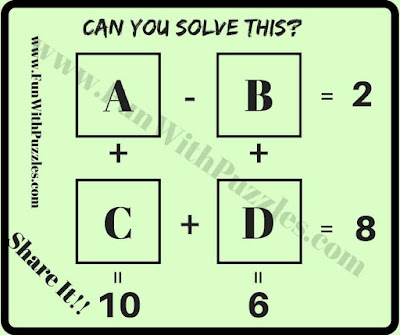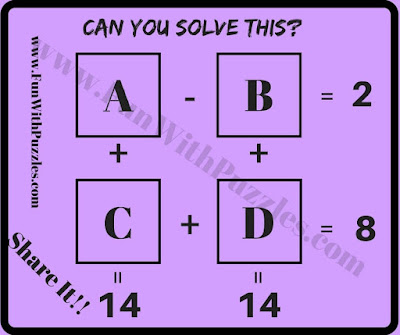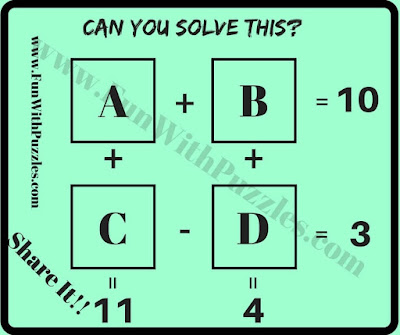## Thursday, February 6, 2020

Mathematical is a very interesting subject. Students will love Maths if this subject is thought in an interesting way. Here we are presenting few Mathematical equation puzzles which are presented in the form of picture puzzles. These are easy Mathematical Questions for students. Let us see how many of these equations you can solve it quickly?
How to Solve Mathematical Puzzle Questions
In each of these Mathematical Puzzle Questions, four Mathematical equations are given as Picture Puzzle. There are four variables in these equations marked as A, B, C and D. Your challenge is to solve these equations and get the values of these variables as quickly as possible.
The answer to these Mathematical Puzzles Questions is given at the end.1. Can you solve these Mathematical Equations?2. Can you find values of A, B, C and D in these equations?3. What are the values of A, B, C and D in these equations?4. Solve these Mathematical Equations5. Will you solve these Mathematical Equations?

Do check out below mentioned Maths Brain Teasers and Puzzles to challenge your brain
List of Mathematical Brain Teasers and Puzzles

1. Math Brain Teasers With Answers: It contains many easy Maths Brain Teasers for Kids and Teens to twist your mind.

2. Math Picture Brain Teasers with Answers and Explanation: It contains different types of Mathematical Brain Teasers presented in the form of Picture Puzzles Images.

3. Middle School Math Brain Teasers: It contains Fun Maths Brain Teasers for Students to challenge your brain.

4. Number Brain Teasers: It contains Mathematical Puzzles in which number equations contains the logical patterns. Your challenge is to find these logical patterns and then find the value of the number which will replace the question mark.

1.puzzle 4 answer is supposed to be: 5 4
3 2

1.2.your answer is right but this answer is also right if a= 6, b=3, c=2 and d=3

2.Fist Puzzle answer is A=5 B=3 C=5 and D=3

1.3.2ND EQUATION A=9 B=2 C=6 D=5 1st EQUATION A=11 B=9 C=3 D=5

1.2nd Equation A=11 B=9 C=3 D=5 this is the proper ans

4.Puzzel 4 ans
4+5=9
4+1=5
8=6

1.A=5,B=3,C=5,D=3

2.Bro plzz give me.solution of 1st puzzle plzzz

5.Answer of 5 is A = 7,B = 3, C=4, D=1

6.7.1.